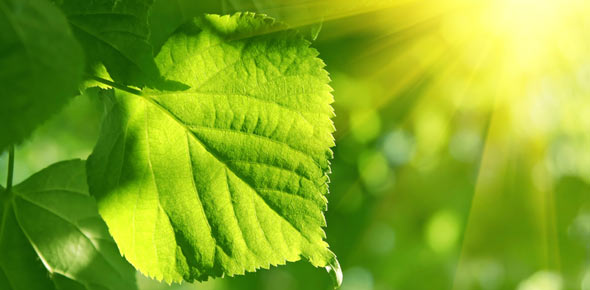# Basic Equation For Photosynthesis

14 Questions | Total Attempts: 344SettingsQuiz on photosynthesis

• 1.
What is the purpose of photosynthesis?
• A.

• B.

To make plants grow

• C.

To create carbohydrates from light energy

• D.

To create ATP for the plant

• 2.
What is the equation for photosynthesis?
• A.

Glucose+oxygen------------> (light energy) CO2+H20

• B.

CO2+H2O-----------> (light energy) gluscose+oxygen

• C.

• 3.
What types of organisms undergo photosynthesis?
• A.

All living things

• B.

Humans

• C.

Bacteria

• D.

Plants

• 4.
What are the two reactions that occur during photosynthesis?
• A.

Krebs cycle and calvin cycle

• B.

ATP and light reactions

• C.

Dark reactions and calvin cycle

• D.

Light reactions and calvin cycle

• 5.
What are the inputs and outputs of light reactions?
• A.

• B.

• C.

There are none

• 6.
How many atp go into the calvin cycle?
• A.

6

• B.

3

• C.

9

• D.

1

• 7.
NADPH turns into what in the Calvin Cycle?
• A.

• B.

• C.

• D.

• 8.
What does PGAL (how many carbons?) turn into?
• A.

4, glucose

• B.

7, carbohydrates

• C.

3, glucose

• D.

3, hydrogen

• 9.
Where does the Light Reactions occur?
• A.

Thylakoids

• B.

Stroma

• C.

Thylakoids and chloroplasts

• D.

All the above

• 10.
Where does the Calvin Cycle occur?
• A.

The entire cell

• B.

Stroma

• C.

The inner membrane of the thylakoids

• D.

Thylakoids

• 11.
What does ATP do?
• A.

Creates carbohyrdrates

• B.

Creates usable energy for cellular work

• C.

Makes photosynthesis

• D.

All the above

• 12.
Whats the equation for ATP?
• A.

• B.

• C.

• D.

A+P+P+P

• 13.
What is the function of NADP+?
• A.

• B.

To create ATP

• C.

To make photosynthesis work

• D.

• 14.
What is the job of the electron transport chain?
• A.

To make proteins

• B.

To make atp synthase

• C.

To transport a system of electrons and to evenually create lots of atp

• D.Back to top